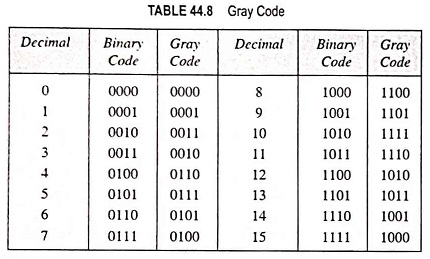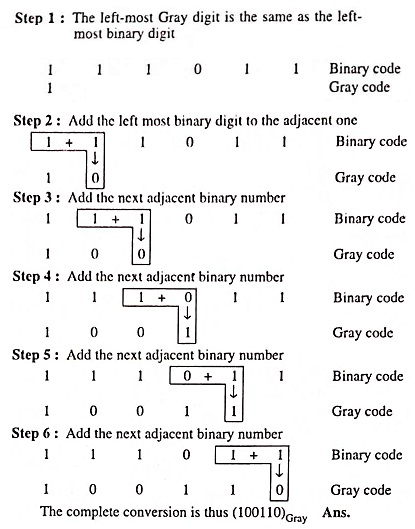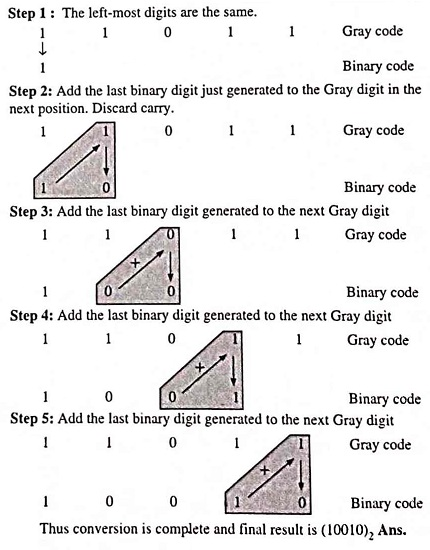## What is Gray code? (Definition, Conversions & Examples):

This Gray code belongs to a class of codes called minimum-change code, in which only one bit in the code group changes when going from one step to the next. This is an unweighted code, which means that there are no specific weights assigned to the bit position. Because of this, the Gray code is not suited for arithmetic operations but finds applications in input/output devices and some types of analog-to-digital converters (ADCs).

The Gray code representation for the decimal numbers 0 through 15, together with the straight binary code is shown in Table 44.8.By examining above Table 44.8 for Gray code groups for each decimal number it can be seen that in going from any one decimal number to the next, only one bit of the Gray code changes. For instance, in going from decimal 3 to 4, the Gray code changes from 0010 to 0110, with only the second bit from the left changing. Going from 14 to 15 the Gray-code bits change from 1001 to 1000 with only the last bit changing. This is the principal characteristic of the Gray code. Because of this feature, an amount of switching is minimized and the reliability of the switching system is improved. This code is used extensively for shaft encoders, where error susceptibility increases when the number of bit changes between adjacent numbers in a sequence.

### Binary-To-Gray Conversion:

For conversion of binary number into a Gray code number, following rules are applied.

1. The most significant digit (left-most digit) in the Gray code is the same as the corresponding digit in the binary number.
2. Going from left to right, add each adjacent pair of binary digits to get the next Gray code digit. Discard carries if any.

Example 44.73: Obtain the following conversion : (111011)binary to Gray code.

Solution:### Gray-To-Binary Conversion:

For conversion of Gray code number into binary code number, the applicable rules are as follows:

1. The most significant digit (left-most) in the binary code is the same as the corresponding digit in the Gray code.
2. Add each binary digit generated to the Gray digit in the next adjacent position. Discard carries if any.

Example 44.74: Convert the Gray Code 11011 to equivalent binary code.

Solution:Scroll to Top